# SQL Server中INNER JOIN与子查询IN的性能测试 - 潇湘隐者 - 博客园`DBCC FREEPROCCACHE;`
`GO`
`DBCC DROPCLEANBUFFERS;`
`GO`

`SET STATISTICS IO ON;`
`SET STATISTICS TIME ON;`

`SELECT  h.* FROM`
`Sales.SalesOrderHeader h`
`WHERE SalesOrderID IN ( SELECT SalesOrderID FROM Sales.SalesOrderDetail)`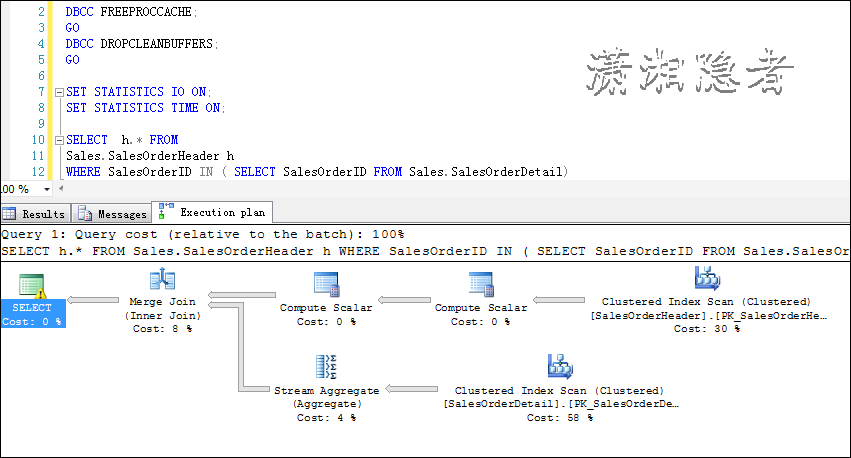`DBCC FREEPROCCACHE;`
`GO`
`DBCC DROPCLEANBUFFERS;`
`GO`
`SET STATISTICS IO ON;`
`SET STATISTICS TIME ON;`

`SELECT h.* FROM Sales.SalesOrderHeader h`
`INNER JOIN Sales.SalesOrderDetail d ON h.SalesOrderID = d.SalesOrderID`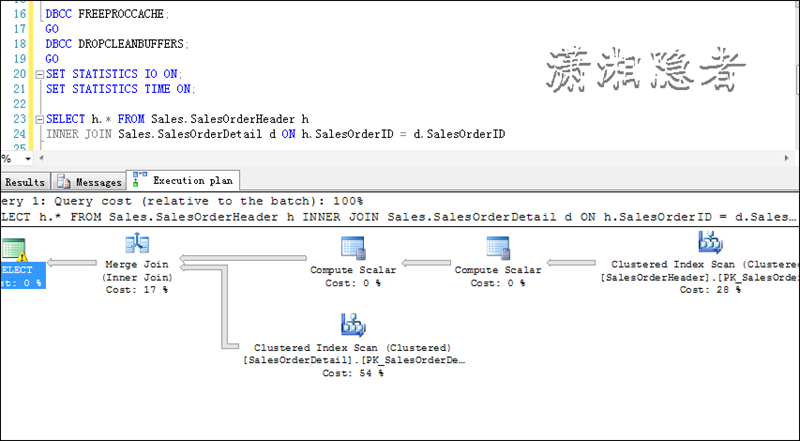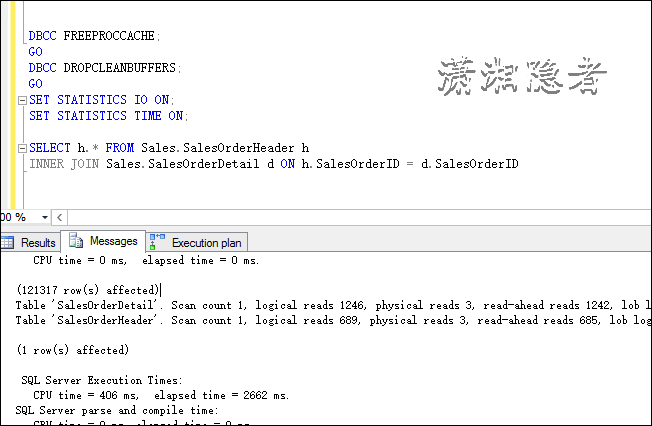`CREATE TABLE P`
`(`
`    PID    INT ,`
`    Pname  VARCHAR(24)`
`)`

`INSERT INTO dbo.P`
`SELECT 1, 'P1' UNION ALL`
`SELECT 2, 'P2' UNION ALL`
`SELECT 3, 'P3'`

`CREATE TABLE dbo.C`
`(`
`    CID       INT ,`
`    PID       INT ,`
`    Cname  VARCHAR(24)`
`)`

`INSERT INTO dbo.c`
`SELECT 1, 1, 'C1' UNION ALL`
`SELECT 2, 1, 'C2' UNION ALL`
`SELECT 3, 2, 'C3' UNION ALL`
`SELECT 3, 3, 'C4'`

`SELECT  h.* FROM`
`Sales.SalesOrderHeader h`
`WHERE SalesOrderID IN ( SELECT SalesOrderID FROM Sales.SalesOrderDetail);`

`SELECT DISTINCT h.* FROM Sales.SalesOrderHeader h`
`INNER JOIN Sales.SalesOrderDetail d ON h.SalesOrderID = d.SalesOrderID;``SET STATISTICS IO ON;`
`SET STATISTICS TIME ON;`

`SELECT  C.*`
`FROM    Sales.Customer C`
`        INNER JOIN Person.Person P ON C.PersonID = P.BusinessEntityID;`

`SELECT  C.*`
`FROM    Sales.Customer C`
`WHERE  C.PersonID IN ( SELECT Person.Person.BusinessEntityID`
`                                     FROM   Person.Person );`

INNER JOIN与子查询IN的实际执行计划对比的百分比为66% VS 34% ， 子查询IN的性能还比 INNER JOIN的性能要好一些. IO几乎无差别，cpu time 与elapsed time的对比情况来看，子查询IN的性能确实要好一些。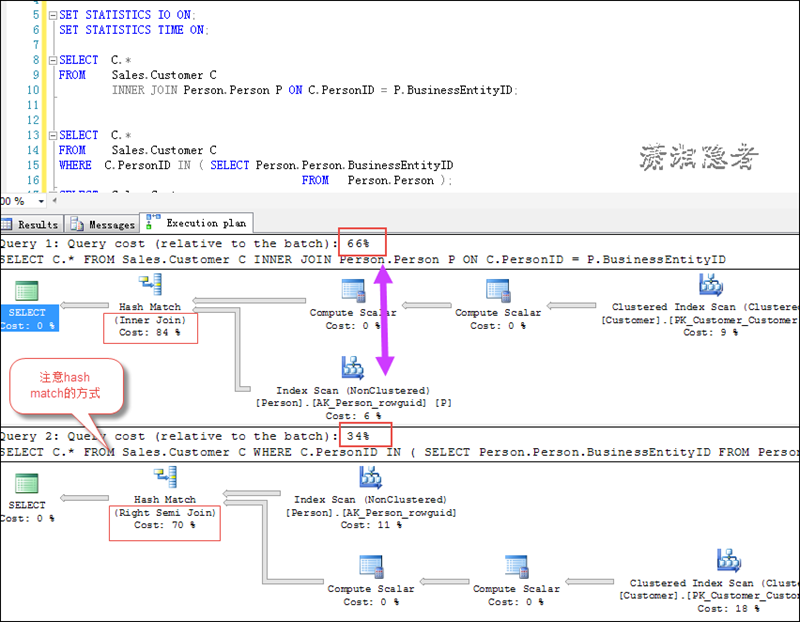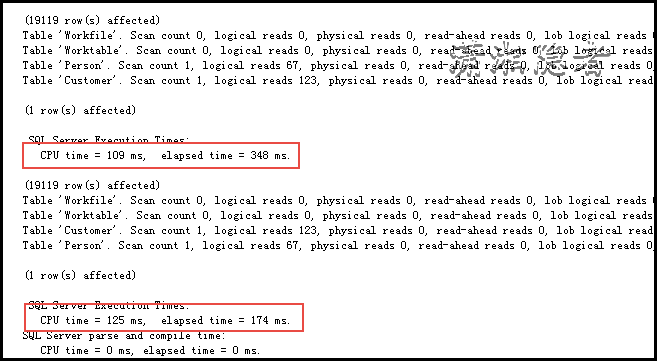`CREATE TABLE P`
`(`
`    P_ID    INT IDENTITY(1,1),`
`    OTHERCOL        CHAR(500),`
`    CONSTRAINT PK_P PRIMARY KEY(P_ID)`
`)`
`GO`

`BEGIN TRAN`
`DECLARE @I INT = 1`
`WHILE @I<=10000`
`BEGIN`
`    INSERT INTO P VALUES (NEWID())`
`    SET @I = @I+1`
`    IF (@I%500)=0`
`    BEGIN`
`        IF @@TRANCOUNT>0`
`        BEGIN`
`            COMMIT`
`            BEGIN TRAN`
`        END`
`    END`
`END`
`IF @@TRANCOUNT>0`
`BEGIN`
`    COMMIT`
`END`
`GO`

`CREATE TABLE C`
`(`
`    C_ID  INT IDENTITY(1,1) ,`
`    P_ID   INT  FOREIGN KEY REFERENCES P(P_ID),`
`    COLN  CHAR(500),`
`    CONSTRAINT PK_C  PRIMARY KEY (C_ID)`
`)`

`SET NOCOUNT ON;`

`DECLARE @I INT = 1`
`WHILE @I<=1000000`
`BEGIN`
`    INSERT INTO C VALUES ( CAST(RAND()*10 AS INT)+1,  NEWID())`
`    SET @I = @I+1`
`END`
`GO`

`SET STATISTICS IO ON;`
`SET STATISTICS TIME ON;`

`SELECT C.* FROM dbo.C C`
`INNER JOIN dbo.P  P ON C.P_ID = P.P_ID`
`WHERE P.P_ID=8`

`SELECT * FROM dbo.C`
`WHERE P_ID IN (SELECT P_ID FROM dbo.P WHERE P_ID=8)`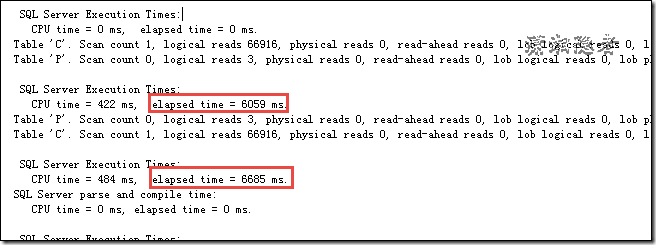`USE [AdventureWorks2014]`
`GO`
`CREATE NONCLUSTERED INDEX [IX_C_N1]`
`ON [dbo].[C] ([P_ID])`
`INCLUDE ([C_ID],[COLN])`
`GO`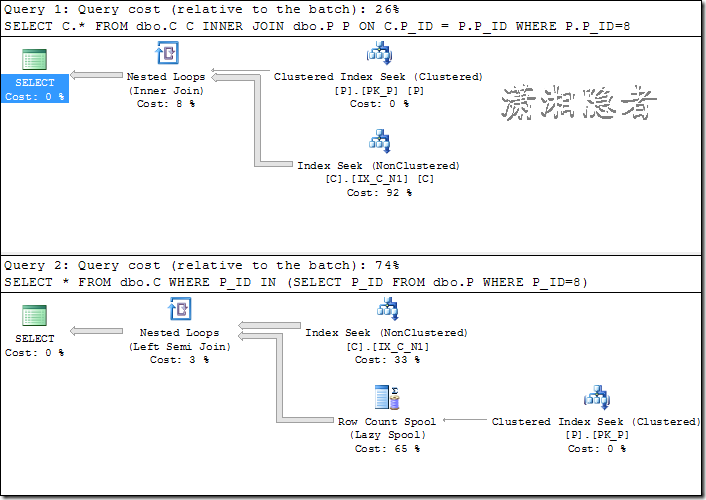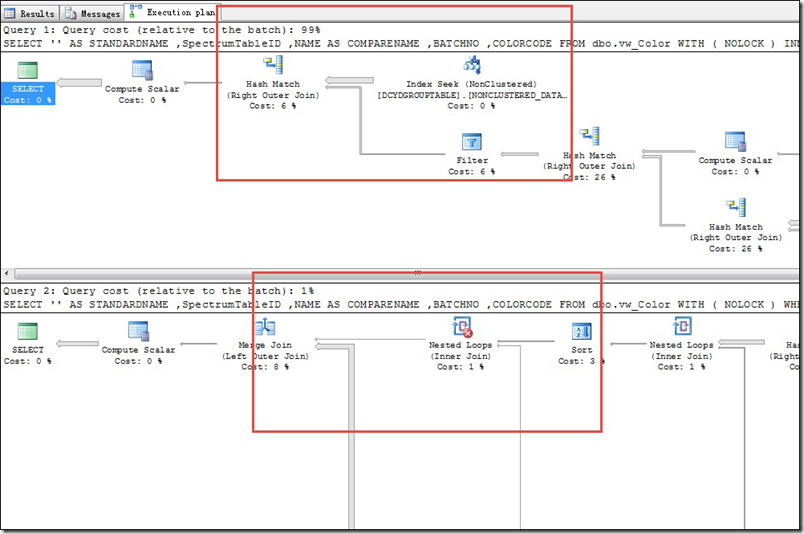### 觉得文章有用就打赏一下文章作者

#### 支付宝扫一扫打赏#### 微信扫一扫打赏• QQ咨询
• 回顶